Get instant live expert help with Excel or Google Sheets“My Excelchat expert helped me in less than 20 minutes, saving me what would have been 5 hours of work!”

#### Post your problem and you’ll get Expert help in seconds.

Your message must be at least 40 characters
Our professional Expert are available now. Your privacy is guaranteed.

# How to Divide in Excel and Google Sheets

Division is one of the most basic operations we have learned growing up. To most people, division is quite difficult. Thanks to Excel, division can now be performed with ease and accuracy.

## How to divide in?

Division in Excel is easily done by using the forward slash “/”, which is the division symbol.

Suppose we want to divide any number (a) by another number (b).  The divide formula is

`=a/b `

where a is the dividend and b is the divisor.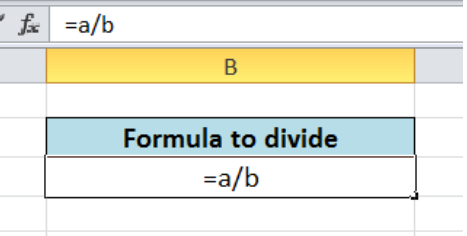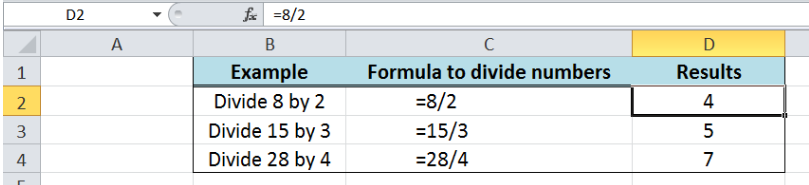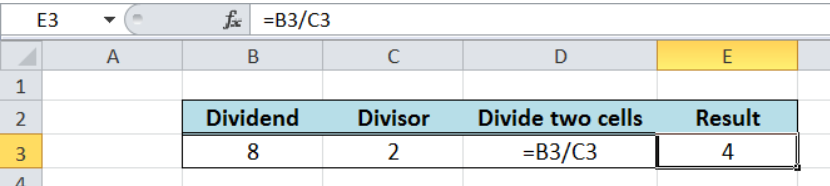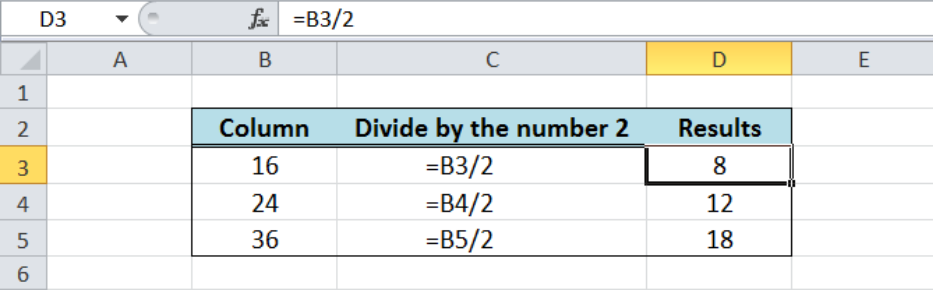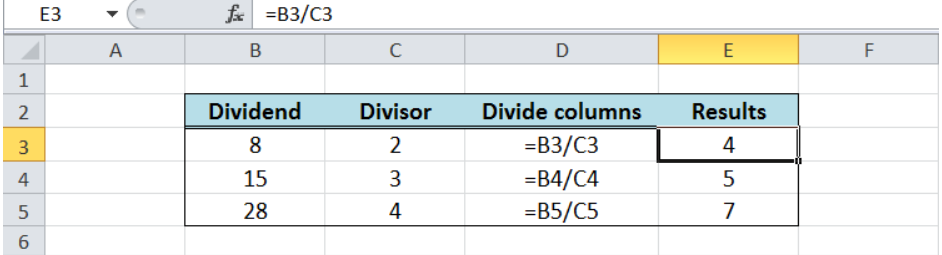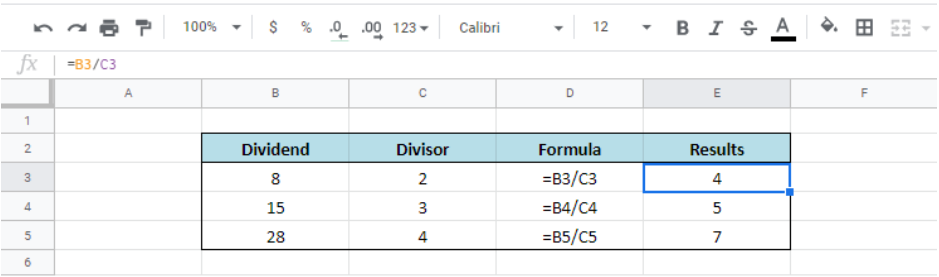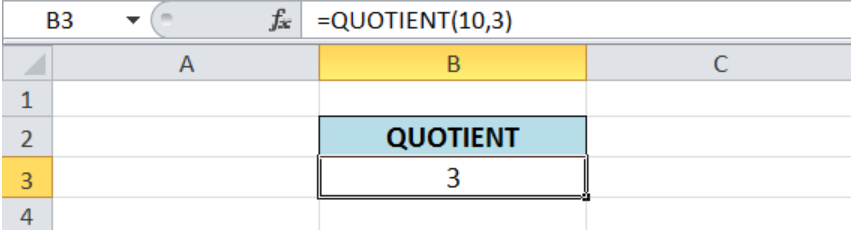Figure 1.  divide formula

## How to divide two numbers?

Dividing is very straightforward.  We enter the equal “=” sign to activate the formula function, then the numerator, followed by the forward slash “/” followed by the denominator.

When we want to divide 8 by 2, the formula to use is:

`=8/2`

where 8 is the numerator or dividend and 2 is the denominator or divisor.

Figure 2.  Divide numbers

## How to divide two cells in?

The formula to divide cells uses  the cell references containing the values as the numerator and denominator.

When we want to divide cell B3 by cell C3, we enter the formula:

`=B3/C3`

Figure 3.  Formula to divide two cells

## How to divide a column by a number?

We can also divide a column by a number.  When we want to divide column B by the number 2, we enter the formula

`=B3/2`

Where the numerator is a cell reference and the denominator is a number.

Figure 4.  Divide a column by a number in Excel

## How to divide columns and Google sheets?

### Divide columns

It is possible to perform division in several columns in as little time as possible.  In order to divide columns, we follow these steps:

• Enter the formula in cell E3: `=B3/C3`
• Press ENTER
• Copy the formula in cell E3 by pressing Ctrl + C, then select cells E4 and E5 and press Ctrl + V to paste the formula

Figure 5.  Divide columns

As a result, the values in column B are divided by the corresponding values in column C.

### Divide columns in Google sheets

The formula to divide columns in Google sheets is similar to Excel.  We simply use the cell references as the numerator and denominator in our division formula.

Figure 6.   Divide columns in Google sheets

## Is there a divide function?

Simply put, there is no function for the division operation.  In order to divide,, we have to use the division symbol in a formula.

There is, however, the QUOTIENT function which returns only the integer portion of any division, excluding the remainder.

Syntax:

`=QUOTIENT(numerator, denominator)`

• Numerator – the dividend
• Denominator  – the divisor

Example:

We want to divide 10 by 3.  Using the QUOTIENT function, we enter the formula

`=QUOTIENT(10,3)`

Figure 7.  QUOTIENT function

The QUOTIENT function does not consider the remainder of the division operation.  Dividing 10 by 3 results to 3 with a remainder of 1. However, the QUOTIENT function only returns the integer part of the result, which is “3”.

Most of the time, the problem you will need to solve will be more complex than a simple application of a formula or function. If you want to save hours of research and frustration, try our live Excelchat service! Our Excel Experts are available 24/7 to answer any Excel question you may have. We guarantee a connection within 30 seconds and a customized solution within 20 minutes.